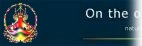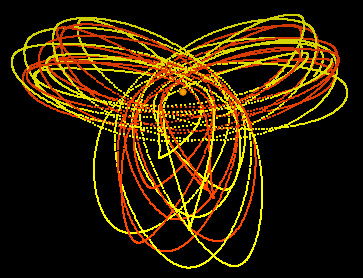Back to Parallels

Back to original reference

C++ code for planet simulation

The following code is part of the CauseF program, Menus and scenarios - Planets.

In particular it serves to create the trajectories of the planets in 3D space, rendered here as their paths:grav_tmp = gravitation;
grav_tmp *= ball_1.mass * ball_array[i].mass;//here the centre is the attractor
if (dist != 0.0f)
grav_tmp *= 1.0f / dist;//force strength is inversely proportional to the distance
gravity_v = subtract_vector(ball_1.pos, ball_array[i].pos);
gravity_v = normalise_vector(gravity_v, &l);
gravity_v = multiply_vector(gravity_v, grav_tmp, 3);